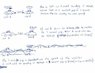# Finding the velocity of a following car with respect to distance between car infront

Dav15
This question is related to adaptive cruise control in new vehicles.
Adaptive cruise control automatically determines the speed at which a vehicle should be traveling by measuring the distance between it, and the car in front (mainly for use on highways)
There is a general rule that three seconds is a safe following distance.
So I suppose that the question is trying to find the acceleration (or deceleration) that the vehicle needs to maintain that gap.
Of course this would cut out when the car reaches a speed, pre determined by the driver (to avoid speeding).

Relevant equations

$$v=u + at$$

The attempt at a solution

I honestly have no clue, I'm not going to bother with posting my attempt because it is so pitiful xD

Last edited:

Bhumble

Welcome to PF. No attempt is worthless because it let's people see where you are going wrong so they can help guide you to an answer. Also a lot of people won't help if you don't at least show some effort.

I'm not sure what exactly your question is either.

Dav15

Thanks Bhumble,
My question is, if the distance between the two cars is known, how would I find the speed the following car should be going to maintain a specific distance (that distance is m/s x 3)

I drew a very rough diagram, to try explain what I am asking :)

EDIT: I apologise for my handwriting :)

#### Attachments

•img042.jpg
39.8 KB · Views: 411
Bhumble

It seems to me that you are interested in the position and with a given distance equivalent to 3 seconds of time.
Are you suppose to be considering acceleration as well?
$s = s_0 + \vec{v} t + \frac{1}{2} a t^2$

Or are we just interested in position and velocity?

Either way the key to setting up the problem is to equate the positions of the two cars when the car in front is at time = t and the following car is time = t+3.

So
$s_a(t=t) = s_b(t=t+3)$
Then it's just some algebra to solve for what you are interested in.

The equation that you put as relevant makes me think you are trying to ask a different question than you illustrated though.